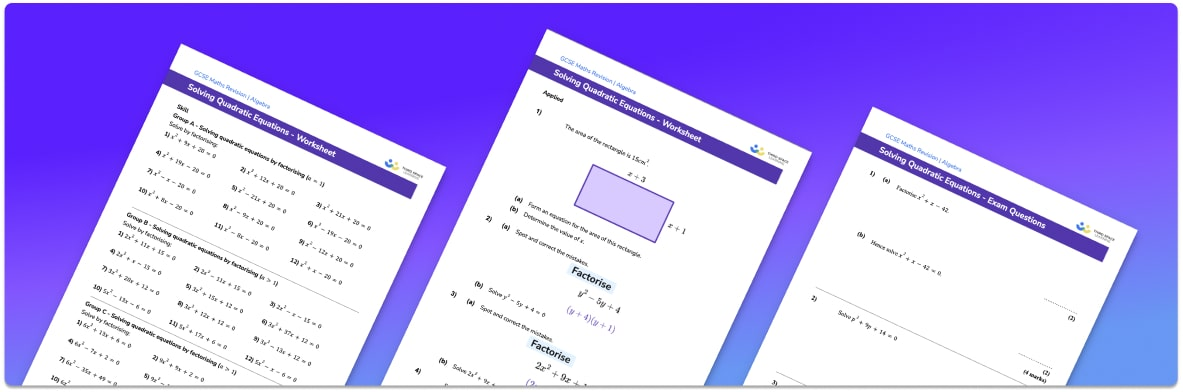• Section 1 of the solving quadratic equations worksheet contains 20+ skills-based solving quadratic equations questions, in 3 groups to support differentiation
• Section 2 contains 3 applied solving quadratic equations questions with a mix of worded problems and deeper problem solving questions
• Section 3 contains 3 foundation and higher level GCSE exam style questions on solving quadratic equations
• Answers and a mark scheme for all solving quadratic equations questions are provided
• Questions follow variation theory with plenty of opportunities for students to work independently at their own level
• All questions created by fully qualified expert secondary maths teachers
• Suitable for GCSE maths revision for AQA, OCR and Edexcel exam boards

• This field is for validation purposes and should be left unchanged.

You can unsubscribe at any time (each email we send will contain an easy way to unsubscribe). To find out more about how we use your data, see our privacy policy.

### Solving quadratic equations at a glance

Quadratic equations are equations where the highest power of x is 2. There are three methods that can be used to solve quadratic equations: factorising, using the quadratic formula or completing the square.

When factorising quadratics or using the formula, it is important to ensure that all terms of the equation are on the left-hand side of the equation, with the right hand side being equal to zero. When solving by completing the square, any terms in x^2 and x must be on the left-hand side of the equation. Quadratic equations usually have two solutions and when solving by completing the square, you must remember to include the positive and negative options when performing the square root to give both solutions.

Solving quadratics is a skill that is used extensively in A Level maths and so any student wanting to progress to A Level should ensure they are confident with these three methods.

The coefficient of a term is the number in front of the power of x e.g. the term 4x^2 has a coefficient of 4, or the coefficient of x^2 is 4.

Looking forward, students can then progress to additional solving equations worksheets and other algebra worksheets, for example a sequences worksheet, simultaneous equations worksheet or straight line graphs worksheet.For more teaching and learning support on Algebra our GCSE maths lessons provide step by step support for all GCSE maths concepts.

## Do you have KS4 students who need more focused attention to succeed at GCSE?There will be students in your class who require individual attention to help them succeed in their maths GCSEs. In a class of 30, it’s not always easy to provide.

Help your students feel confident with exam-style questions and the strategies they’ll need to answer them correctly with our dedicated GCSE maths revision programme.

Lessons are selected to provide support where each student needs it most, and specially-trained GCSE maths tutors adapt the pitch and pace of each lesson. This ensures a personalised revision programme that raises grades and boosts confidence.

Find out more